LearnWorkPlayGames ™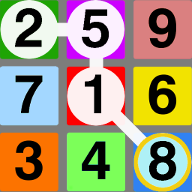Numbino Make the longest sum chains in a changing number grid as quickly as you can! Numbino is an easy-to-learn, math puzzler where the goal is to find simple, long sum equations in a changing number grid. This game provides challenge and fun for players of all ages!

Play!

Choose a platform to play on:

Video

 VIDEO DEMO AND TUTORIAL

Screenshots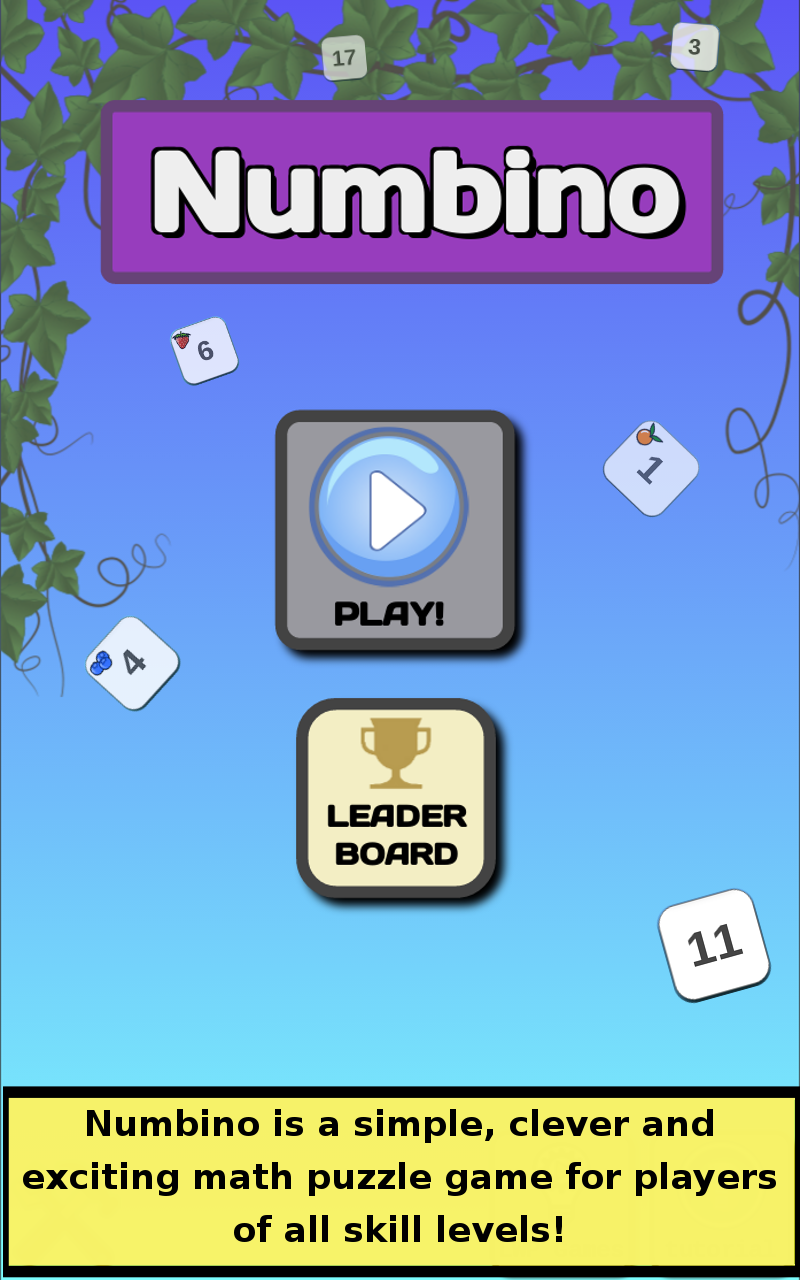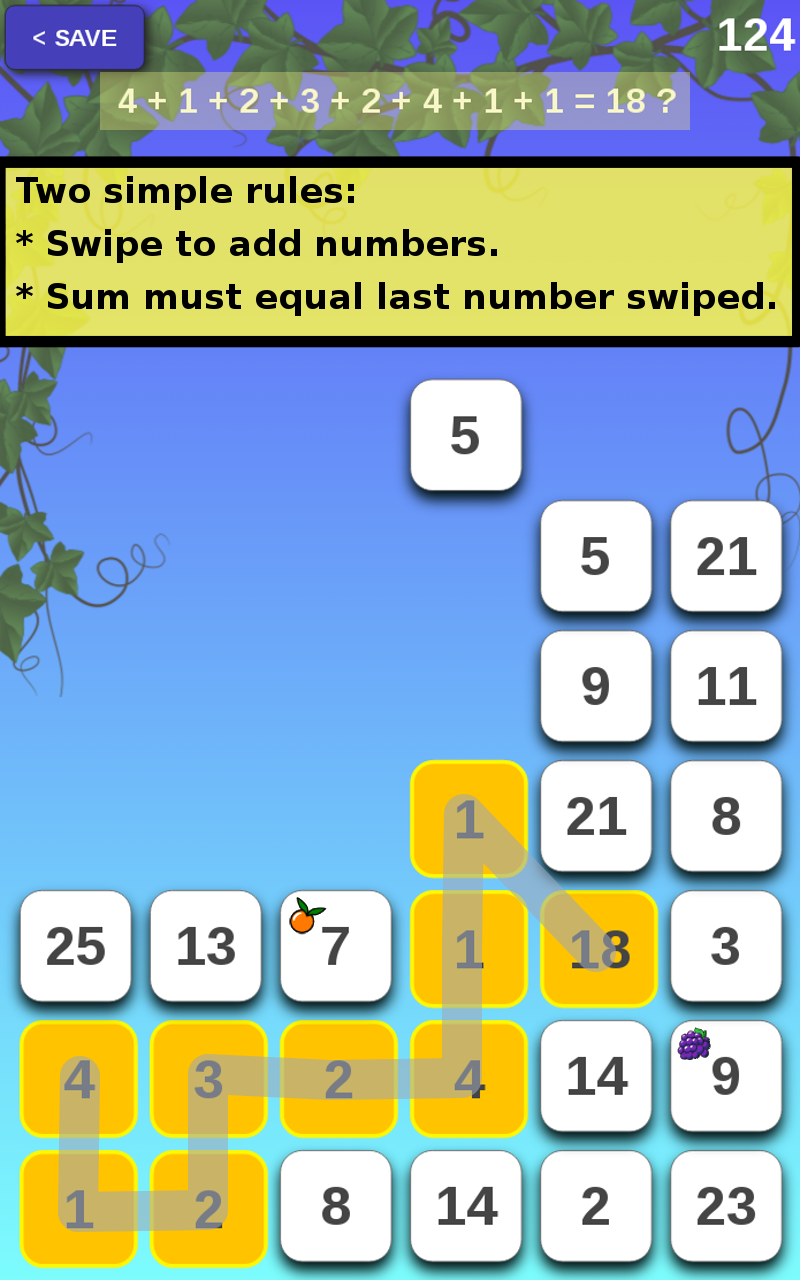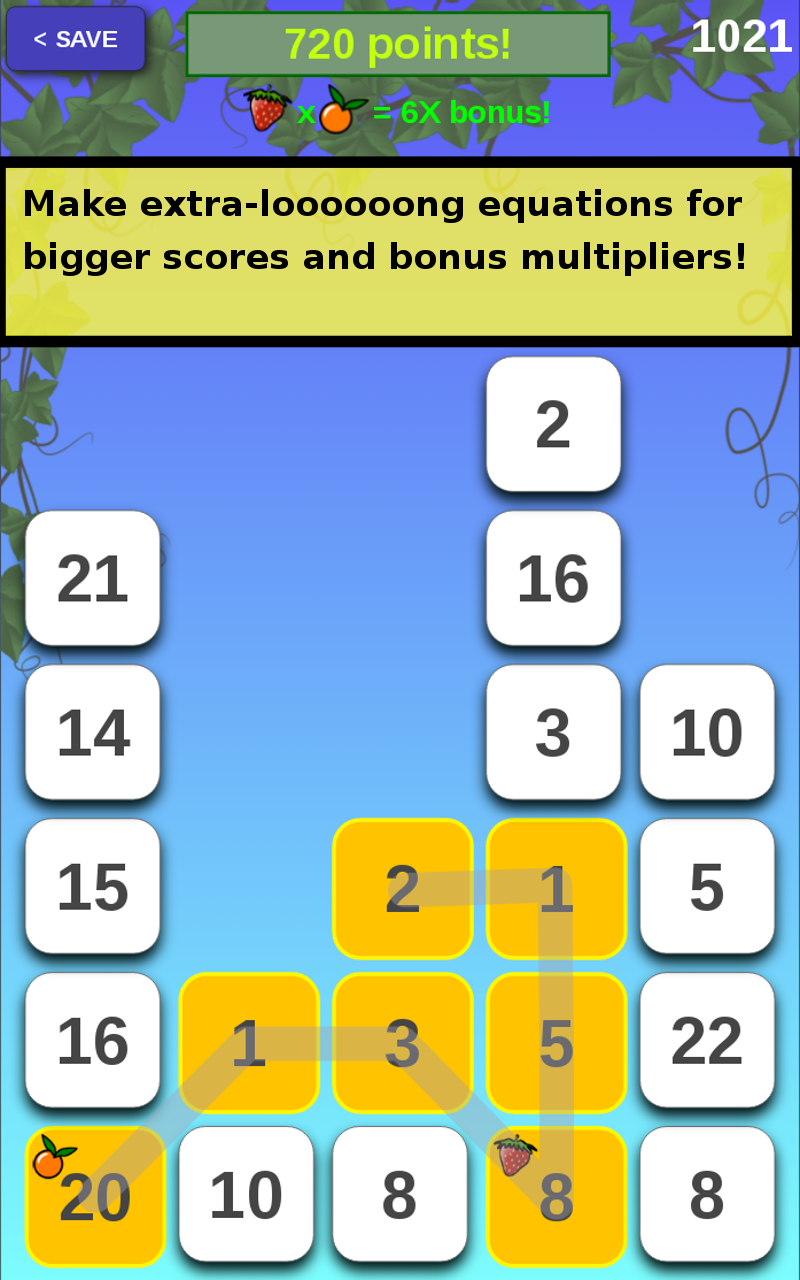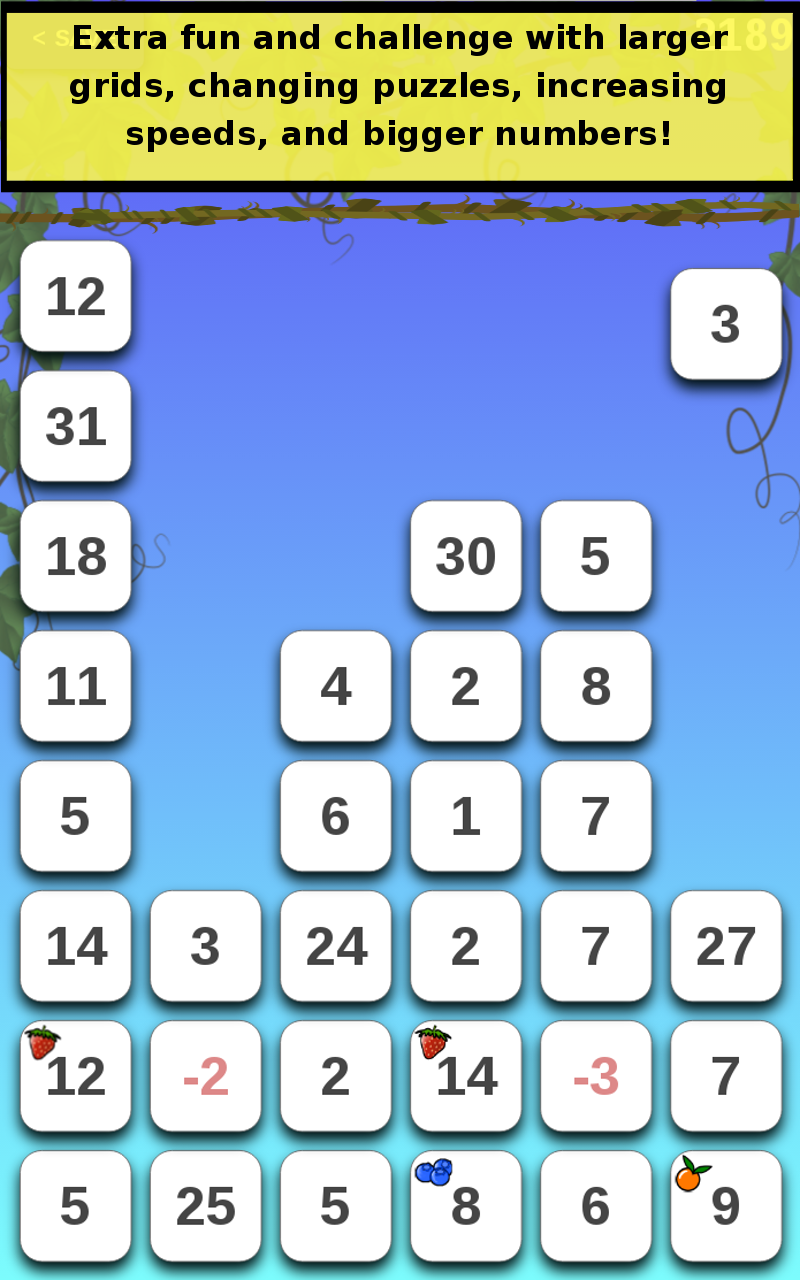Description

• Swipe to add numbers.

• Sum must equal last number swiped.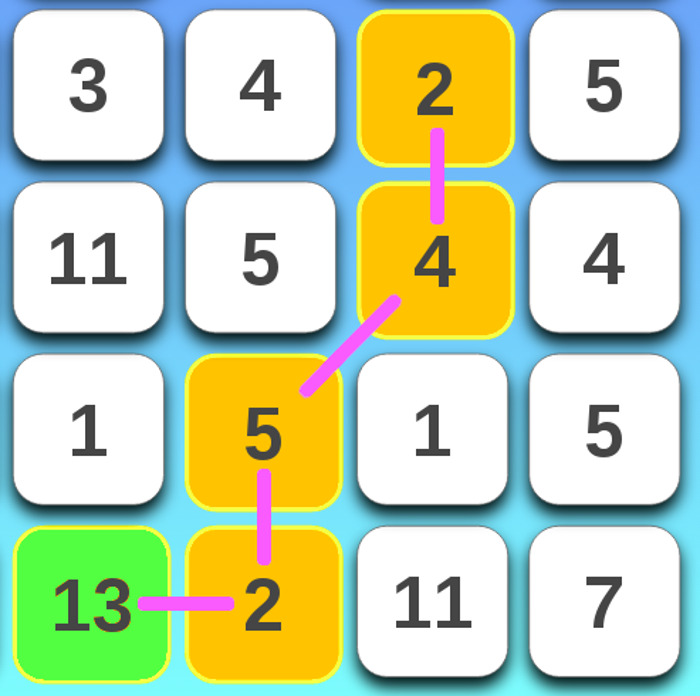(EXAMPLE: 2 + 4 + 5 + 2 = 13)

• Make sums quickly to clear numbers before the grid fills up!

• As you progress, the game will get harder:

=> bigger numbers
=> negative numbers
=> faster speeds

• TIP: Make loooong equations:

=> bigger scores
=> bonus multipliers

• MORE TIPS:

• If a cell has a bonus multiplier, you can tap it once and spend it to get a new random number for that cell.

• Try to keep a good mix of different sized numbers to ensure you can always make an equation.

• Score = (sum) X (equation length) X (bonuses)
•  About   |   Terms of Use   |   Privacy Policy